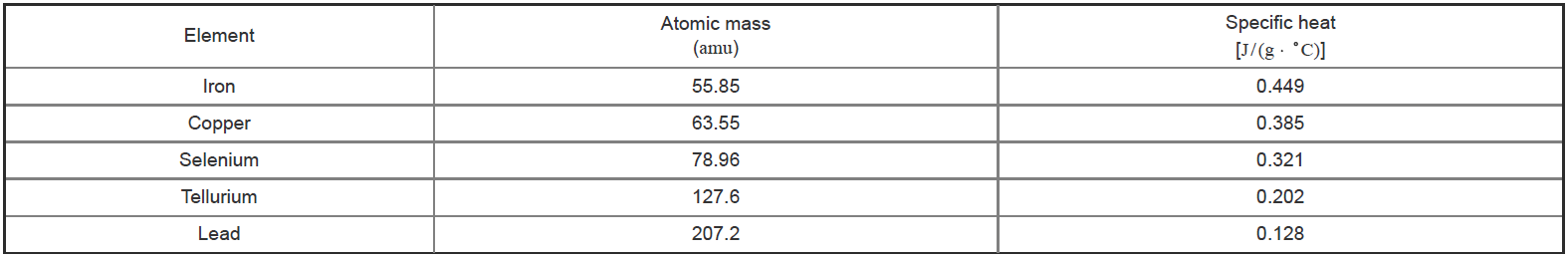# Problem: Using the equation y = 25x + 0.015, where y represents specific heat and x represents the inverse of atomic mass, calculate the atomic mass of an element with a measured specific heat of 0.24 J/ (g ⋅ °C).Express your answer in atomic mass units to two significant figures.Based on specific heat measurements, Pierre Dulong and Alexis Petit proposed in 1818 that the specific heat of an element is related to its atomic weight (atomic mass). Thus, by measuring the specific heat of a new element, its atomic weight could be readily established. The specific heat and atomic mass for a series of elements are provided for reference.

###### FREE Expert Solution
90% (87 ratings)View Complete Written Solution
###### Problem Details

Using the equation y = 25x + 0.015, where y represents specific heat and x represents the inverse of atomic mass, calculate the atomic mass of an element with a measured specific heat of 0.24 J/ (g ⋅ °C).